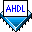## Using Case Statement Logic

 See AlsoThe decoder.tdf file shown below is a 2-bit-to-4-bit decoder. It converts two binary code inputs into a "one-hot" code.

```SUBDESIGN decoder
(
code[1..0]	: INPUT;
out[3..0]	: OUTPUT;
)
BEGIN
CASE code[] IS
WHEN 0 => out[] = B"0001";
WHEN 1 => out[] = B"0010";
WHEN 2 => out[] = B"0100";
WHEN 3 => out[] = B"1000";
END CASE;
END;
```

In this example, the input group `code[1..0]` has the value `0`, `1`, `2`, or `3`. The equation following the appropriate `=>` symbol in the Case Statement is activated. For example, if `code[]` is `1`, `out1` is set to `B"0010"`. Since the values of the expression are all different, only one `WHEN` clause can be active at one time.